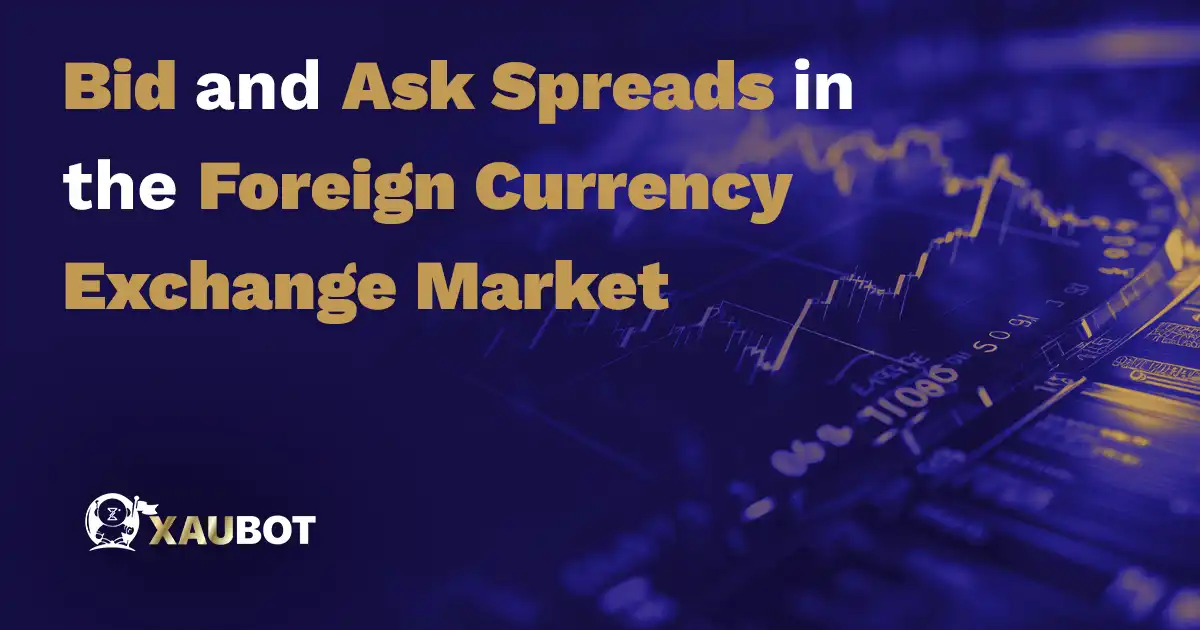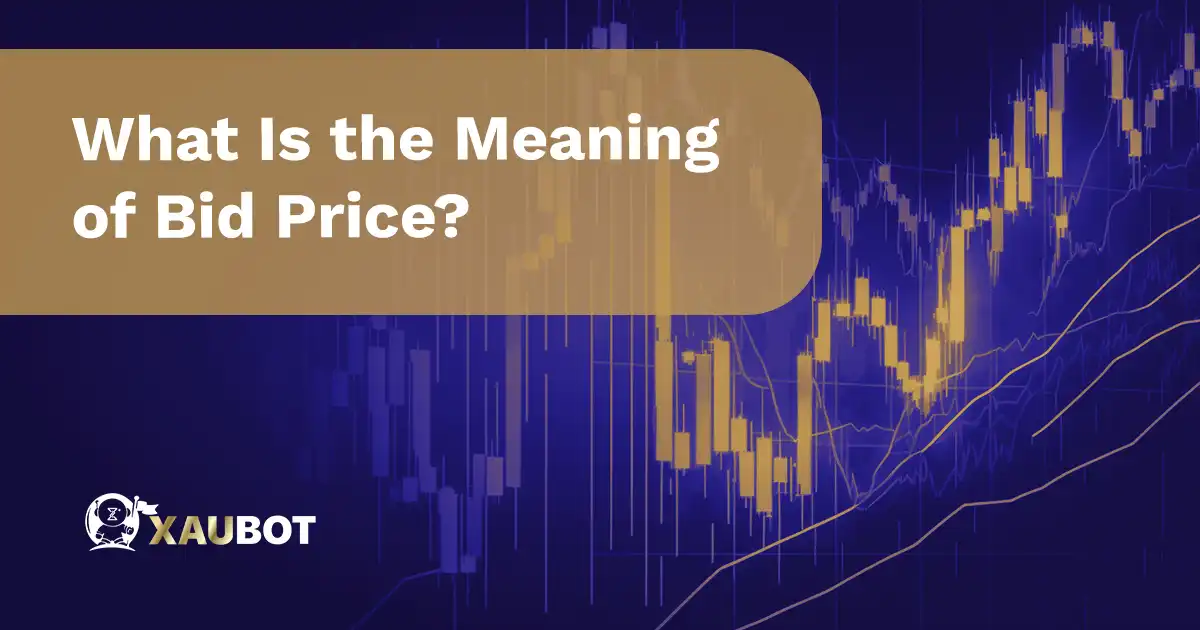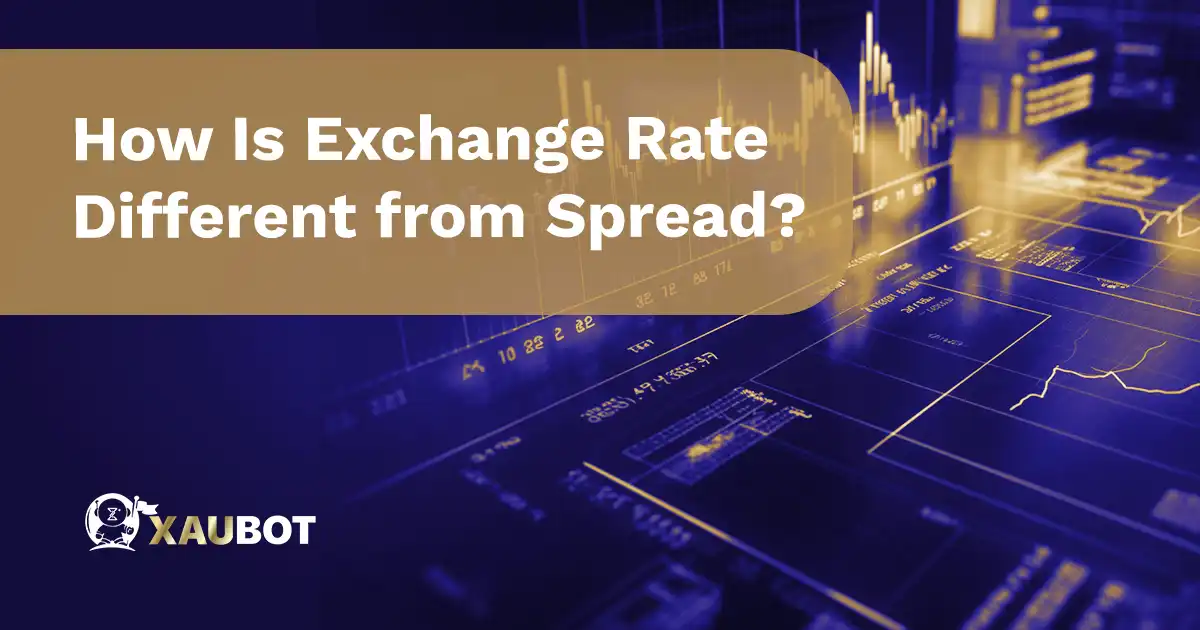Bid and Ask Spreads in the forex - XAUBOTContents

One of the terms that you might hear a lot in forex trading is the term spread. Of course there are different types of spreads available in various markets. In its essence, spread means difference between two values. These two values could be prices or percentages and rates.

One form of spread that is especially prevalent in the forex market is the bid-ask spread. Many believe that this is one of the most important factors to keep in mind while trading and that it counts heavily toward your final profits.

So let’s take a look at the bid-ask spread and see how important it is in forex trading.

The bid and ask spread can be defined simply as the difference between the price you pay to buy an asset and the price you receive for selling it. Naturally in the forex market this would refer to the difference in the price you give to buy a currency pair and the price for selling it.

It is mainly through this difference in prices where traders can make profits trading in the forex market. Though keep in mind that depending on the currency pair, the spread can be smaller or much larger.

But the spread itself is made of two very important notions – namely bid price and ask price. We are also going to take a look at them.

But before doing so, let’s have a quick example of what a bid-ask spread looks like.

For this example, we can consider the trading pair made from the United States dollar and the Japanese Yen. Together they form the pair USD/JPY.

Suppose that at this moment the quoted price for this pair is as follows:

USD/JPY = 142

This means that in order to purchase one unit of the base currency, i.e. the US dollar, you need to pay 142 units of the quoted currency, i.e. the Japanese Yen.

Now, if the price goes from what has been mentioned above to USD/JPY = 144, we can see that the quoted price for each unit of the base currency has increased by 2 units. In other words, there is a difference of 2 units of the quoted currency in this pair for its bid and ask prices. This is the ask-bid spread for the trading pair USD/JPY in our example.

But to further understand the role and significance of bid-ask spread in forex, you need to know the two separate parts of the spread – which are the bid price and ask price.

## What Is the Meaning of Bid Price?What Is the Meaning of Bid Price

The bid price is the price considered from the perspective of the buyer. This is why sometimes they define the bid price as the buy price. Basically, it is the price that the buyer is going to pay for an asset or a financial instrument in the market.

By and large, the bid price is not as high as the ask price. And that is exactly how the difference between these two prices makes the bid-ask spread.

A market maker such as your forex broker will normally make most of its money as the broker through this way, which means through the bid-ask spread.

## What Is the Meaning of Ask Price?

On the other hand, we have the ask price which is the exact opposite of a bid price. The ask price is the price at which the seller wants to sell an asset or a financial instrument to the buyer. For this reason, the ask price is sometimes interchangeably used with the sell price or the offer price.

Naturally, the ask price is always more than the bid price, since the seller will define the ask price but the bid price is the price the buyer will pay and usually does not play a role in it. Even if the buyer does get to have a say in the bid price, that would turn it into an unsolicited bid.

If you do not know the importance of the bid-ask spread in the forex market, you simply cannot be active in this market.

The reason is that as we mentioned at the beginning, the bid and ask prices are the prices related to buying and selling a currency. So these are directly the prices you pay in the market for your position.

This is why you always have to be monitoring the spreads in the market. But what is important is that depending on the broker, you might get a little bit of a different spread on a pair than what you would get in another broker.

This is because brokers also make their money from the spread in the buy and sell prices.

Furthermore, you need to know that the bid-ask spread is normally defined in terms of pips. A pip is the smallest unit of price change. And it is equal to one change in the fourth decimal digit in the quoted price for any currency pair. Naturally, each pip is the same in degree, but in terms of monetary value, it would depend on the lot size or the position size and also the quoted currency in the pair, which is the second currency.

## How Is Exchange Rate Different from Spread?How Is Exchange Rate Different from Spread

A term that is usually mistaken for the bid-ask spread is the exchange rate. But exchange rate and the bid-ask spread are actually two different concepts.

To clarify any confusion, let us take a quick look at what the exchange rate is and how it relates to the forex market.

So the concept of exchange rate itself is the rate or price that exists in an exchange for the trade of one currency to another currency. Naturally, this rate of exchange between these two currencies is crucially important and will impact the outcome of the trade

So for example in our example above we mentioned the quoted price for the currency pair USD/JPY and we also said that this quoted price is equal to 142. This quoted price in this example can also be considered as the exchange rate for the pair US dollar and Japanese Yen.

It means that in order to exchange these two currencies to one another, for every US dollar you need 142 Japanese Yens, and vice versa.

The exchange rate is mostly affected by fundamental indicators that are related to the economy as a whole and it is less so impacted by the smaller and short term price movements.

Economic factors including supply and demand of the currency and other economic factors that are directly related to the currency, such as the interest rate set by the central bank of that currency can also shift the value of the exchange rate.

## Conclusion

The bid and ask spread is one of the most important concepts that every forex trader ought to know. It refers to the difference between the bid price (or the buy price – the price that the buyer pays) and the ask price (or the sell and offer price – the price that the seller wants). The figure can play the ultimate role in the profits that you can make from forex trading.

Share Posts
You may also like